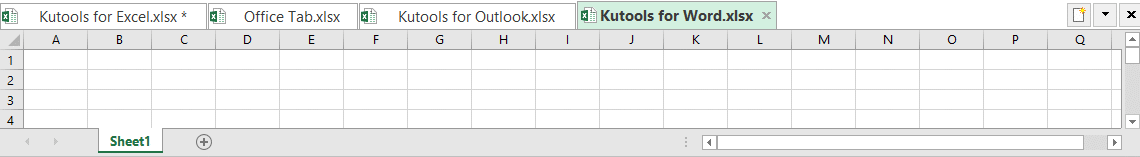## How to Save and Use Your VBA Macros in All Workbooks in Excel?

In some cases, you may need to use one VBA macro multiple times in the future. Is it possible to save someway the VBA module to any new document so it will be available in all workbooks? The answer is yes. In this tutorial, we will introduce an easy way to accomplish your goal.Save and Use the VBA Code in All Workbooks

#### Save and Use the VBA Code in All Workbooks

For example, you want to use the VBA code to convert numbers to English words and save the VBA module in all workbooks in case you want to use the VBA code in the future. Please do as follows.

1. Press the Alt + F11 keys in Excel, and it opens the Microsoft Visual Basic for Applications window.

2. Click Insert > Module and paste the following macro in the Module Window.

VBA code: Convert numbers to words

``````Function NumberstoWords(ByVal MyNumber)
'Update by Extendoffice
Dim xStr As String
Dim xFNum As Integer
Dim xStrPoint
Dim xStrNumber
Dim xPoint As String
Dim xNumber As String
Dim xP() As Variant
Dim xDP
Dim xCnt As Integer
Dim xResult, xT As String
Dim xLen As Integer
On Error Resume Next
xP = Array("", "Thousand ", "Million ", "Billion ", "Trillion ", " ", " ", " ", " ")
xNumber = Trim(Str(MyNumber))
xDP = InStr(xNumber, ".")
xPoint = ""
xStrNumber = ""
If xDP > 0 Then
xPoint = " point "
xStr = Mid(xNumber, xDP + 1)
xStrPoint = Left(xStr, Len(xNumber) - xDP)
For xFNum = 1 To Len(xStrPoint)
xStr = Mid(xStrPoint, xFNum, 1)
xPoint = xPoint & GetDigits(xStr) & " "
Next xFNum
xNumber = Trim(Left(xNumber, xDP - 1))
End If
xCnt = 0
xResult = ""
xT = ""
xLen = 0
xLen = Int(Len(Str(xNumber)) / 3)
If (Len(Str(xNumber)) Mod 3) = 0 Then xLen = xLen - 1
Do While xNumber <> ""
If xLen = xCnt Then
xT = GetHundredsDigits(Right(xNumber, 3), False)
Else
If xCnt = 0 Then
xT = GetHundredsDigits(Right(xNumber, 3), True)
Else
xT = GetHundredsDigits(Right(xNumber, 3), False)
End If
End If
If xT <> "" Then
xResult = xT & xP(xCnt) & xResult
End If
If Len(xNumber) > 3 Then
xNumber = Left(xNumber, Len(xNumber) - 3)
Else
xNumber = ""
End If
xCnt = xCnt + 1
Loop
xResult = xResult & xPoint
NumberstoWords = xResult
End Function
Function GetHundredsDigits(xHDgt, xB As Boolean)
Dim xRStr As String
Dim xStrNum As String
Dim xStr As String
Dim xI As Integer
Dim xBB As Boolean
xStrNum = xHDgt
xRStr = ""
On Error Resume Next
xBB = True
If Val(xStrNum) = 0 Then Exit Function
xStrNum = Right("000" & xStrNum, 3)
xStr = Mid(xStrNum, 1, 1)
If xStr <> "0" Then
xRStr = GetDigits(Mid(xStrNum, 1, 1)) & "Hundred "
Else
If xB Then
xRStr = "and "
xBB = False
Else
xRStr = " "
xBB = False
End If
End If
If Mid(xStrNum, 2, 2) <> "00" Then
xRStr = xRStr & GetTenDigits(Mid(xStrNum, 2, 2), xBB)
End If
GetHundredsDigits = xRStr
End Function
Function GetTenDigits(xTDgt, xB As Boolean)
Dim xStr As String
Dim xI As Integer
Dim xArr_1() As Variant
Dim xArr_2() As Variant
Dim xT As Boolean
xArr_1 = Array("Ten ", "Eleven ", "Twelve ", "Thirteen ", "Fourteen ", "Fifteen ", "Sixteen ", "Seventeen ", "Eighteen ", "Nineteen ")
xArr_2 = Array("", "", "Twenty ", "Thirty ", "Forty ", "Fifty ", "Sixty ", "Seventy ", "Eighty ", "Ninety ")
xStr = ""
xT = True
On Error Resume Next
If Val(Left(xTDgt, 1)) = 1 Then
xI = Val(Right(xTDgt, 1))
If xB Then xStr = "and "
xStr = xStr & xArr_1(xI)
Else
xI = Val(Left(xTDgt, 1))
If Val(Left(xTDgt, 1)) > 1 Then
If xB Then xStr = "and "
xStr = xStr & xArr_2(Val(Left(xTDgt, 1)))
xT = False
End If
If xStr = "" Then
If xB Then
xStr = "and "
End If
End If
If Right(xTDgt, 1) <> "0" Then
xStr = xStr & GetDigits(Right(xTDgt, 1))
End If
End If
GetTenDigits = xStr
End Function
Function GetDigits(xDgt)
Dim xStr As String
Dim xArr_1() As Variant
xArr_1 = Array("Zero ", "One ", "Two ", "Three ", "Four ", "Five ", "Six ", "Seven ", "Eight ", "Nine ")
xStr = ""
On Error Resume Next
xStr = xArr_1(Val(xDgt))
GetDigits = xStr
End Function
``````

3. Click the Save icon in the top-left corner of the ribbon or click Ctrl + S to open the Save As window.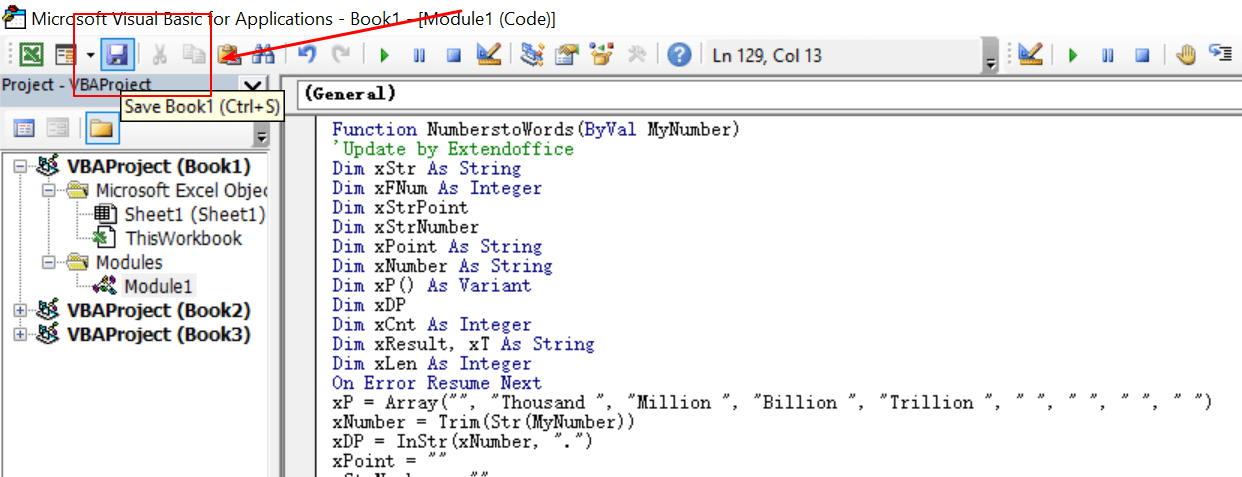4. In the Save As window, input the workbook name in the File name box. And select the Excel Add-in (*.xlam) option in the Save as type drop-down list.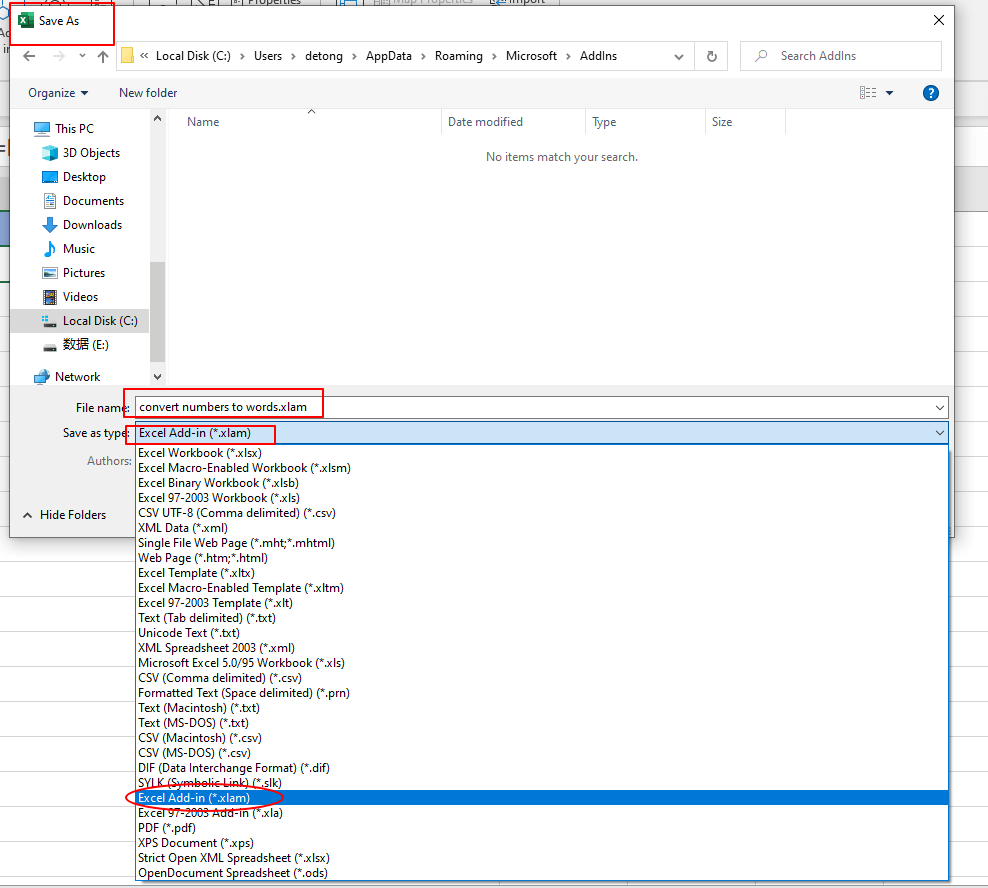5. Then click the Save button to save the workbook with VBA code as an Excel Add-in.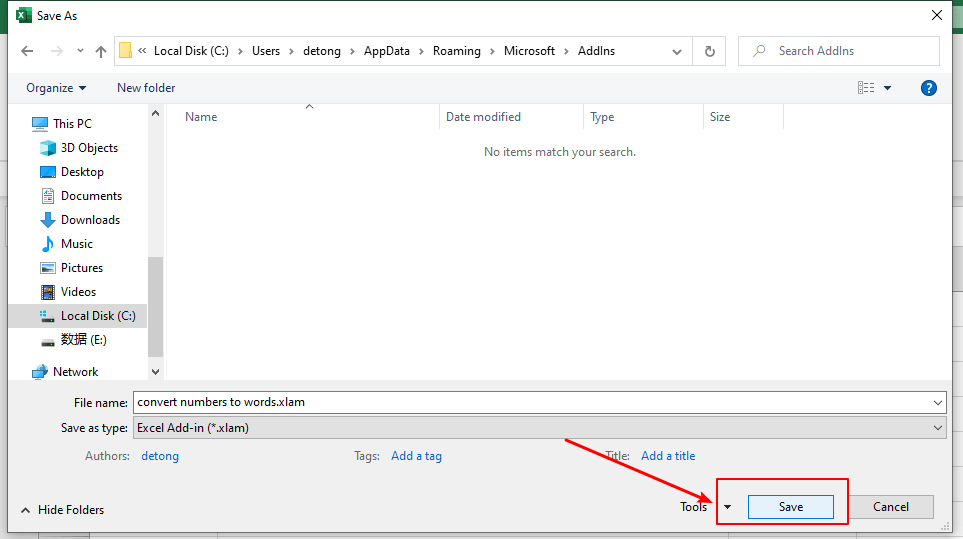6. Back to the Excel, close the empty workbook which has been saved as an Excel Add-in.

7. Open a new workbook with data needed to be converted. Input the formula =NumberstoWords(A2) in cell B2. The #NAME? error value will be returned because VBA code has not been applied in all workbooks yet.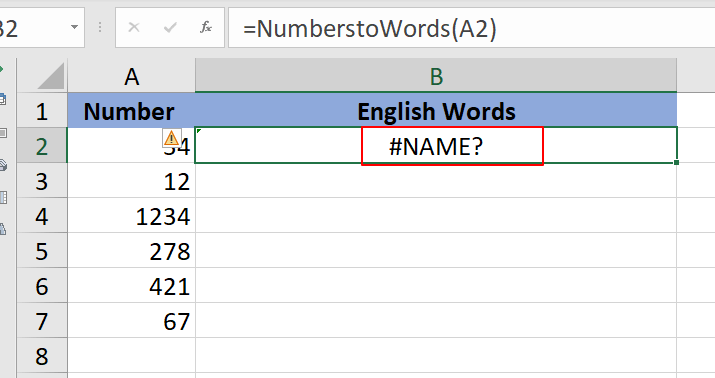8. Go to the Developer tab, click Excel Add-ins in the Add-ins group.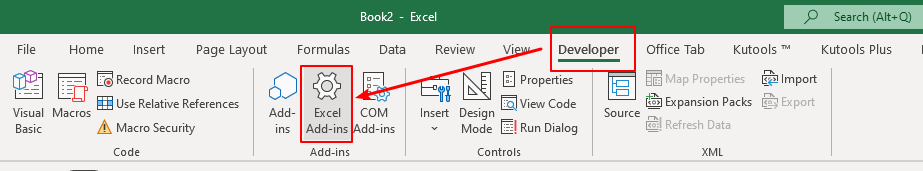9. The Add-in dialog box pops up. Click the Browse button.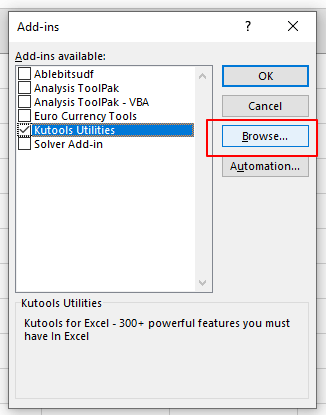10. Choose the Add-in you just saved, then click the OK button.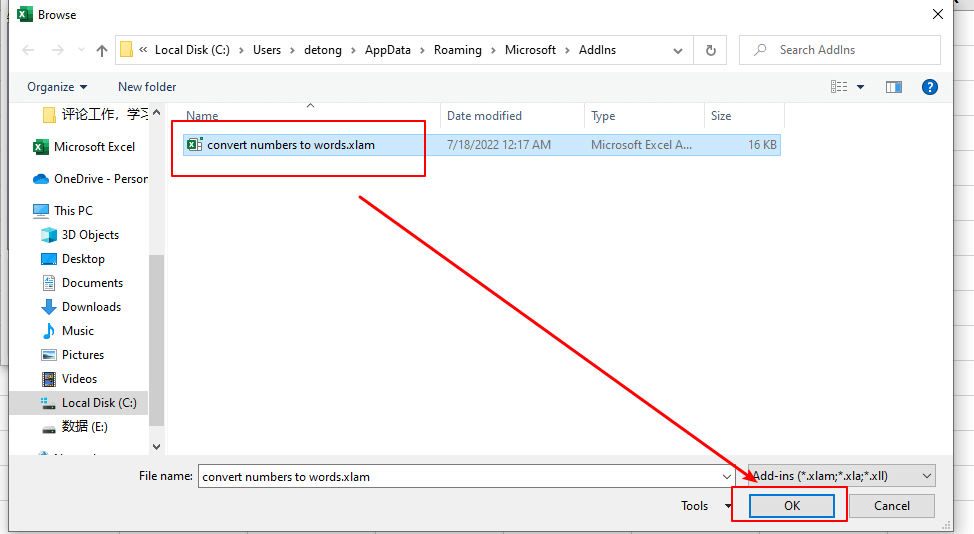11. Then the Convert Number To Words Add-in you customized is inserted and turned on. Click the OK button to finish the setting.12. Now when you input the formula =NumberstoWords(A2) in cell B2 and press the Enter key, the corresponding English words will be returned. Drag the autofill handle down to get all the results.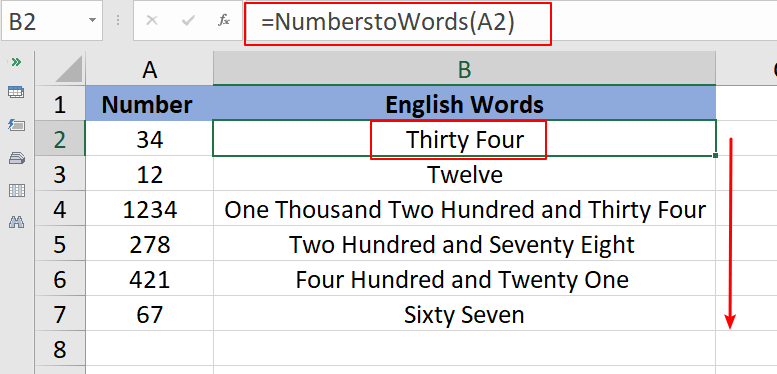Notes:

If you need to run the code manually, there is no way to find it from the above steps. Please don’t worry. There are two ways to run the code.

1. You can add code to the Quick Toolbar and run the code each time the code button is clicked on the toolbar.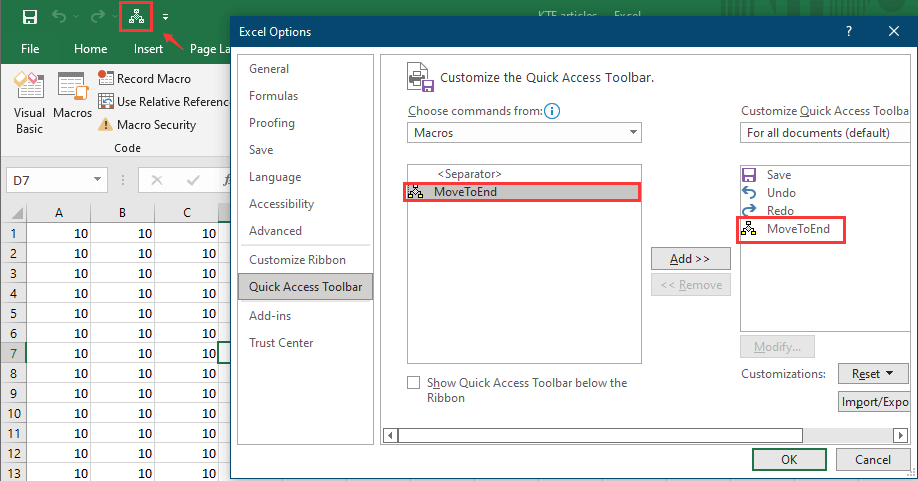2. You can also directly press Alt + F11 to open the code operation box, find the code, and press F5 to run.

#### Other Operations (Articles)

A VBA Code To List All Add Ins In Excel
In Excel, you may add or insert some add ins for better dealing with data. As we know, we can go to the Options window to view all add ins, but is there any way to list all add ins in a sheet? Now, in this tutorial, it provides a VBA code for listing all add ins in Excel.

How To Run VBA Macro When Open Or Close Workbook?
In this article, I will tell you how to run the VBA code while opening or closing the workbook every time.

How To Protect / Lock VBA Code In Excel?
Just like you can use password to protect workbooks and worksheets, you can also set a password for protecting the macros in Excel.

How To Use Time Delay After Running A VBA Macro In Excel?
In some cases, you may need to make a timer delay for triggering a VBA Macro in Excel. For example, when clicking to run a specified macro, it will take effect after 10 seconds. This article will show you a method to achieve it.

## The Best Office Productivity Tools

### Kutools for Excel Solves Most of Your Problems, and Increases Your Productivity by 80%

• Super Formula Bar (easily edit multiple lines of text and formula); Reading Layout (easily read and edit large numbers of cells); Paste to Filtered Range...
• Merge Cells/Rows/Columns and Keeping Data; Split Cells Content; Combine Duplicate Rows and Sum/Average... Prevent Duplicate Cells; Compare Ranges...
• Select Duplicate or Unique Rows; Select Blank Rows (all cells are empty); Super Find and Fuzzy Find in Many Workbooks; Random Select...
• Exact Copy Multiple Cells without changing formula reference; Auto Create References to Multiple Sheets; Insert Bullets, Check Boxes and more...
• Favorite and Quickly Insert Formulas, Ranges, Charts and Pictures; Encrypt Cells with password; Create Mailing List and send emails...
• Extract Text, Add Text, Remove by Position, Remove Space; Create and Print Paging Subtotals; Convert Between Cells Content and Comments...
• Super Filter (save and apply filter schemes to other sheets); Advanced Sort by month/week/day, frequency and more; Special Filter by bold, italic...
• Combine Workbooks and WorkSheets; Merge Tables based on key columns; Split Data into Multiple Sheets; Batch Convert xls, xlsx and PDF...
• Pivot Table Grouping by week number, day of week and more... Show Unlocked, Locked Cells by different colors; Highlight Cells That Have Formula/Name...### Office Tab - brings tabbed interface to Office, and make your work much easier

• Enable tabbed editing and reading in Word, Excel, PowerPoint, Publisher, Access, Visio and Project.
• Open and create multiple documents in new tabs of the same window, rather than in new windows.
• Increases your productivity by 50%, and reduces hundreds of mouse clicks for you every day!